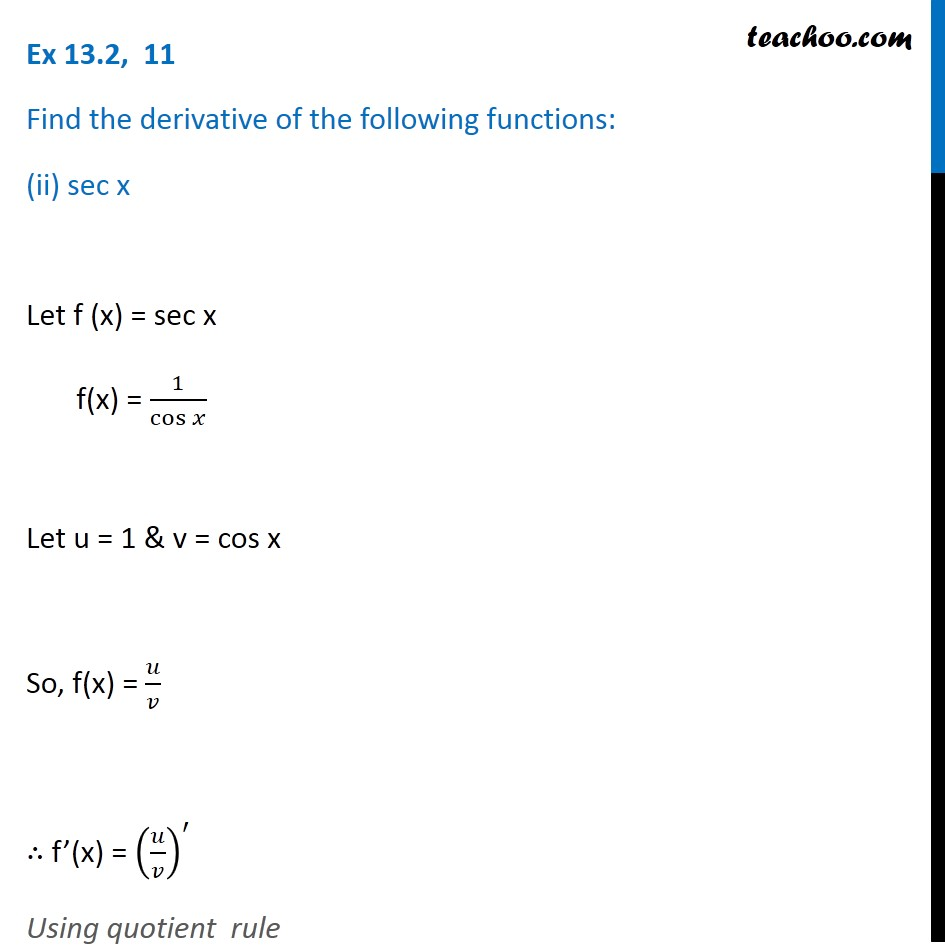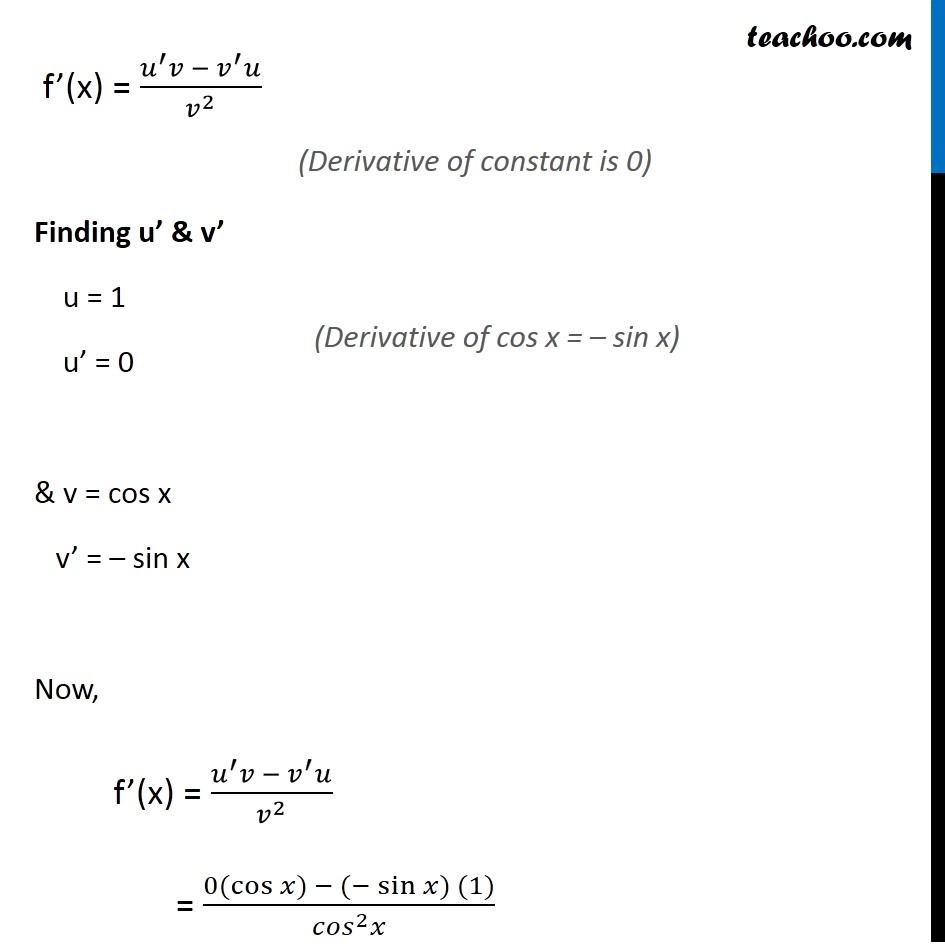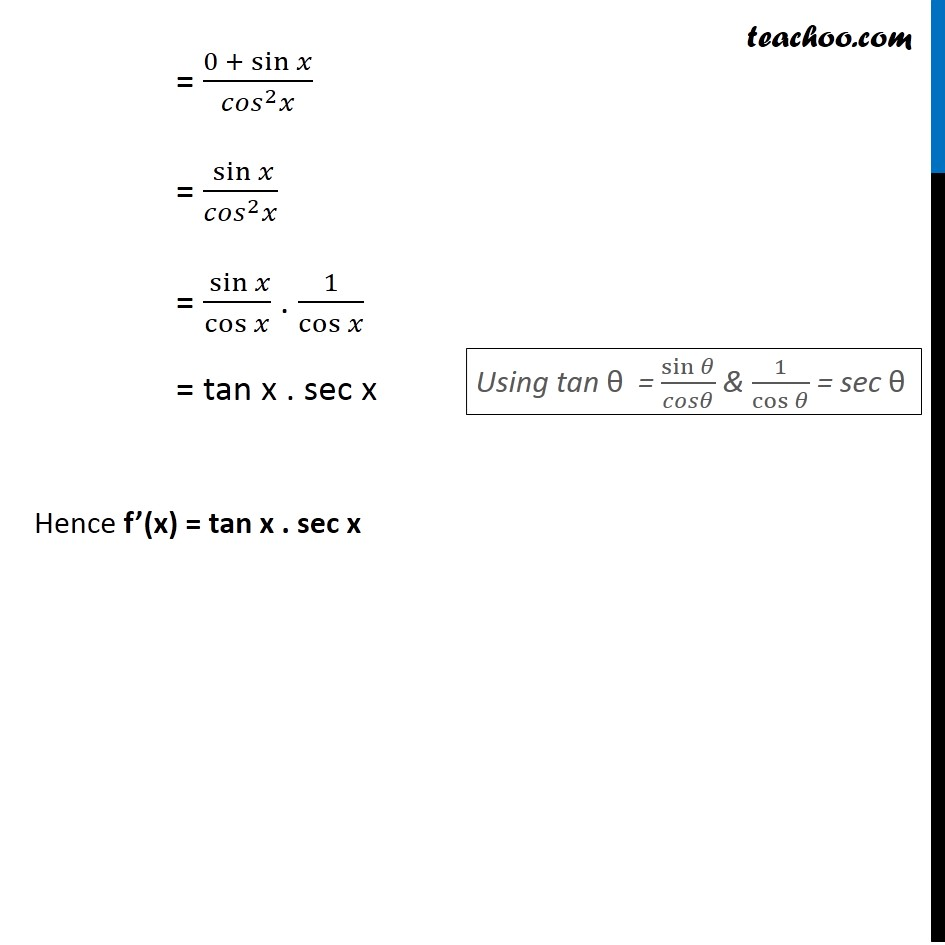Ex 12.2

Chapter 12 Class 11 Limits and Derivatives
Serial order wiseLearn in your speed, with individual attention - Teachoo Maths 1-on-1 Class

### Transcript

Ex 12.2, 11 Find the derivative of the following functions: (ii) sec x Let f (x) = sec x f(x) = 1/cos⁡𝑥 Let u = 1 & v = cos x So, f(x) = 𝑢/𝑣 ∴ f’(x) = (𝑢/𝑣)^′ Using quotient rule f’(x) = (𝑢^′ 𝑣 − 𝑣^′ 𝑢)/𝑣^2 Finding u’ & v’ u = 1 u’ = 0 & v = cos x v’ = – sin x Now, f’(x) = (𝑢^′ 𝑣 − 𝑣^′ 𝑢)/𝑣^2 = (0(cos⁡〖𝑥) − (−sin⁡〖𝑥) (1)〗 〗)/(〖𝑐𝑜𝑠〗^2 𝑥) (Derivative of constant is 0) (Derivative of cos x = – sin x) = (0 +〖 sin〗⁡𝑥)/(〖𝑐𝑜𝑠〗^2 𝑥) = 〖 sin〗⁡𝑥/(〖𝑐𝑜𝑠〗^2 𝑥) = 〖 sin〗⁡𝑥/cos⁡𝑥 . 1/cos⁡𝑥 = tan x . sec x Hence f’(x) = tan x . sec x Using tan θ = sin⁡𝜃/𝑐𝑜𝑠𝜃 & 1/cos⁡𝜃 = sec θ